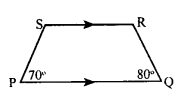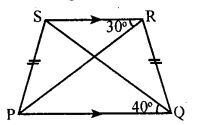# KSEEB Solutions for Class 8 Maths Chapter 15 Quadrilaterals Ex 15.2

In this chapter, we provide KSEEB SSLC Class 8 Maths Chapter 15 Quadrilaterals Ex 15.2 for English medium students, Which will very helpful for every student in their exams. Students can download the latest KSEEB SSLC Class 8 Maths Chapter 15 Quadrilaterals Ex 15.2 pdf, free KSEEB SSLC Class 8 Maths Chapter 15 Quadrilaterals Ex 15.2 pdf download. Now you will get step by step solution to each question.

### Karnataka State Syllabus Class 8 Maths Chapter 15 Quadrilaterals Ex 15.2

Question 1.
In a trapezium PQRS, PQ || RS and ∠E= 70° and Q = 80° calculate the measure of ∠S and ∠R.∠P + ∠S = 180°
[adjacent angles correspondingto parallel sides 70° + ∠S = 180°]
∠S = 180 – 70
∠S = 110°
∠R + ∠Q = 180°
[adjacent angles corresponding to parallel sides ∠R + 80° = 180°]
∠R = 180 – 80
∠R = 100°

Question 2.
In trapezium ABCD, AB || CD. It is given that AD is not parallel to BC. Is ∆ABC ≅ ∆ADC ? Give reasons.
∆ ABC and ∆ ADC are not congruent. It is not possible to show that they are congruent to each other by using any of the four congruence postulates.

Question 3.
In the figure, PQRS is an isosceles trapezium. ∠SRP = 30° and ∠POS = 40°. Calculate the angles ∠RPO and∠RSO.PQ || RS
∠PRS = ∠RPQ = 30° [Alternate angles]
∠RPQ = 30°
∠RSQ = ∠PQS = 40° [Alternate angles]
∠RSQ = 40°

All Chapter KSEEB Solutions For Class 8 maths

—————————————————————————–

All Subject KSEEB Solutions For Class 8

*************************************************

I think you got complete solutions for this chapter. If You have any queries regarding this chapter, please comment on the below section our subject teacher will answer you. We tried our best to give complete solutions so you got good marks in your exam.

If these solutions have helped you, you can also share kseebsolutionsfor.com to your friends.

Best of Luck!!